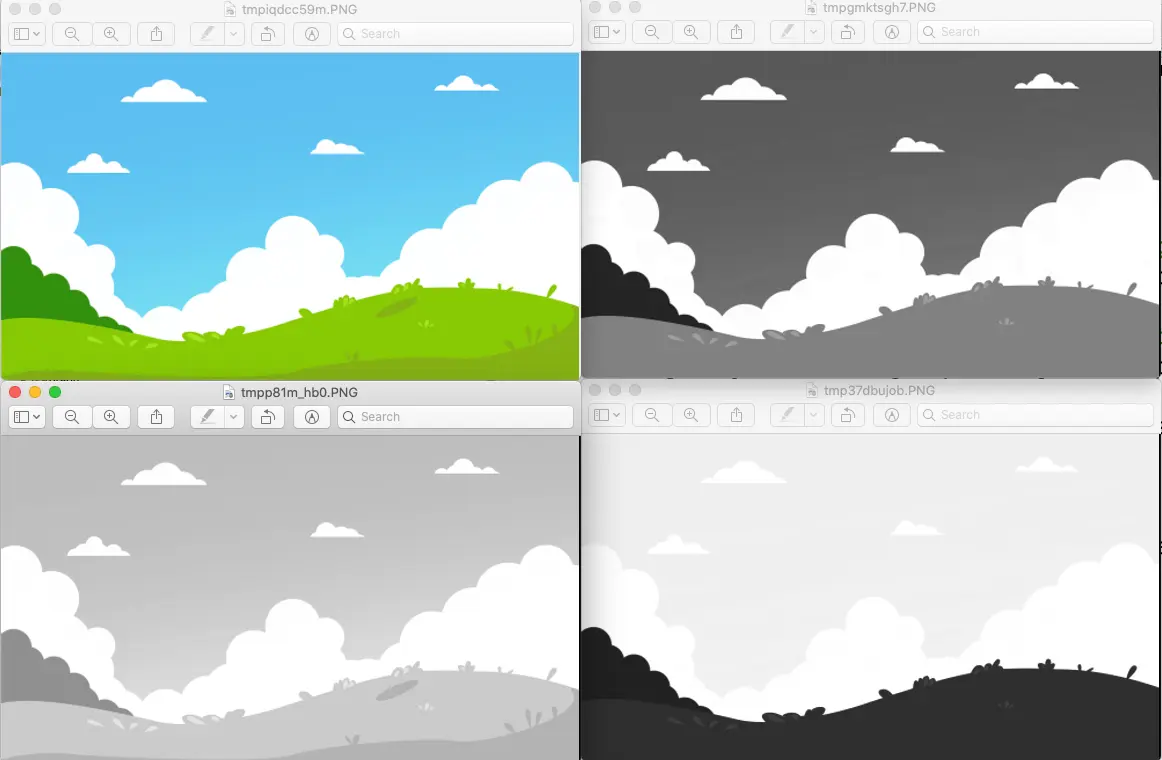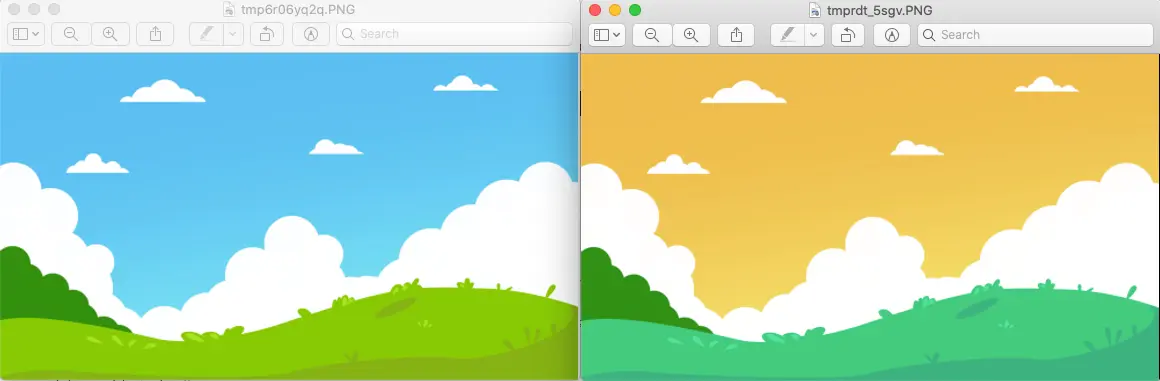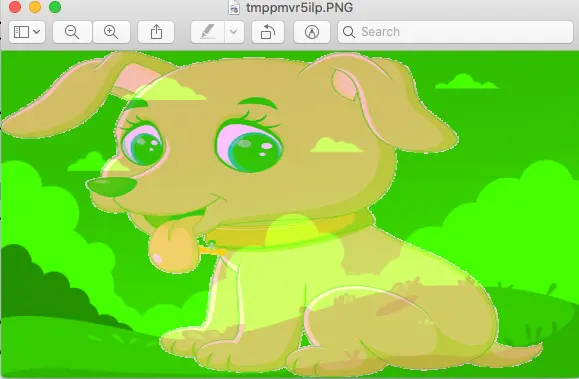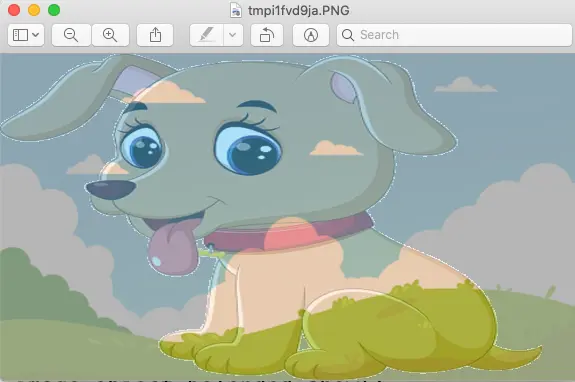# How To Split, Merge & Blend Images Use Python Pillow

A digital image is composed of many pixels, the pixel is the basic unit of the image, and each pixel can use a different color, finally showing a colorful image. The split and merge of images refers to the split and merge of image colors. Python pillow Image class provides methods ( split() and merge() ) for splitting and merging images. This article will show you how to use them with examples.

### 1. Split Image Use split() Method Example.

1. The use of the split() method is relatively simple, this method is mainly used to separate color channels.
2. Below is the example source code.
```# this function will split an image color channel and create it's red, green, and blue channel image objects.

from PIL import Image

def split_image():

image_file_path = '../../../../img/background-image-blue-sky-1.png'

image_object = Image.open(image_file_path)

red_chanel_image_object, green_chanel_image_object, blue_chanel_image_object, alpha_value = image_object.split()

image_object.show()

red_chanel_image_object.show()

green_chanel_image_object.show()

blue_chanel_image_object.show()

print('alpha_value = ', alpha_value)

if __name__ == '__main__':

split_image()

```
3. When you run the above source code, you will get the below picture. The top left image is the original image.### 2. Merge Image Use merge() Method Example.

1. The merge() method provided by the Image class can implement the merge operation of images.
2. Note that image merging can be merging a single image or merging more than two images.
3. The syntax of the merge() method is as follows, this function returns a new Image object.
```Image.merge(mode, bands)

mode: specifies the mode of the output image.

bands: the parameter type is a tuple or a list, and its element value is the color channel that composes the image. For example, RGB represents three color channels, which can be expressed as (r, g, b).```
4. Let us introduce the two types of image merging separately.

#### 2.1 Merge Single Image.

1. The merging of a single image refers to recombining the color channels to get a different image effect.
2. Below is the example source code.
```from PIL import Image

def merge_single_image():

image_file_path = '../../../../img/background-image-blue-sky-1.png'

source_image_object = Image.open(image_file_path)

# Split color channel to create three Image objects.
red_chanel_image_object, green_chanel_image_object, blue_chanel_image_object, alpha_value = source_image_object.split()

# Recombine the color channels image objects and return a new Image object.
merged_image_object = Image.merge('RGB',(blue_chanel_image_object, green_chanel_image_object, red_chanel_image_object))

source_image_object.show()

merged_image_object.show()

if __name__ == '__main__':

merge_single_image()```
3. Below is the resulting image of the above source code. The left image is the original image.#### 2.2 Merge Multiple Images.

1. The merging operation of two images is not complicated, but the mode and size of the two images must be the same, otherwise, they cannot be combined.
2. Therefore, for those images with different modes and sizes, preprocessing is required.
3. Below is the example source code.
```from PIL import Image

def merge_multiple_images():

image1_file_path = '../../../../img/background-image-blue-sky-1.png'
image_object_1 = Image.open(image1_file_path)

image2_file_path = '../../../../img/dog.png'
image_object_2 = Image.open(image2_file_path)

# because the two images's format are the same, we only need to make the 2 images same size.
image_object_3 = image_object_2.resize(image_object_1.size)

# split the above 2 image objects.
r1, g1 , b1, a1 = image_object_1.split()
r2, g2 , b2, a2 = image_object_3.split()

# merge the 2 images use different RGB channel image objects.
image_object_merged = Image.merge('RGB',[r2,g1,b2])

# show the merged image.
image_object_merged.show()

if __name__ == '__main__':

merge_multiple_images()```
4. When you run the above source code, it will show the below image.### 3. Blend Image Use blend() Method Example.

1. The Image class also provides the blend() method to mixing images in RGBA mode (PNG format). The syntax of the function is as follows.
2. Image.blend(image1, image2, alpha).
```image1, image2: represents two Image objects.

alpha: indicates transparency, the value range is 0 to 1, when the value is 0, the output image is equivalent to a copy of image1, and when the value is 1, it is a copy of image2, only when the value is 0.5, the output image is the mix of image1 and image2. So this value determines how well the two images are blended.```
3. Below is the example source code.
```from PIL import Image

# blend 2 images and set the alpha value.
def blend_images():

image1_file_path = '../../../../img/background-image-blue-sky-1.png'
image_object_1 = Image.open(image1_file_path)

image2_file_path = '../../../../img/dog.png'
image_object_2 = Image.open(image2_file_path)

image_object_resized = image_object_2.resize(image_object_1.size)

# set the alpha value to 0.5.
alpha_value = 0.5
image_object_belended = Image.blend(image_object_1, image_object_resized, alpha_value)

image_object_belended.show()

if __name__ == '__main__':

blend_images()```
4. When you run the above python source code, you can get the below image.This site uses Akismet to reduce spam. Learn how your comment data is processed.